MORE IN Mechanics of Materials
VTU Mechanical Engineering (Semester 3)
Mechanics of Materials
June 2015
Total marks: --
Total time: --
INSTRUCTIONS
(1) Assume appropriate data and state your reasons
(2) Marks are given to the right of every question
(3) Draw neat diagrams wherever necessary

1 (a) State Hooke's law and define Poisson's ratio.
3 M
1 (b) Explain stress-strain diagram for mild steel with salient features.
7 M
1 (c) A member ABCD is subjected to point loads P1, P2, P3 & P4 as shown in fig.Q1(c). Calculate the force P2 necessary for equilibrium. If P1=45 kN, P3=450kN & P4=130kN. Determine stresses in each member also determine the total elongation of the member assuming the E to be 2.1×105 N/mm2.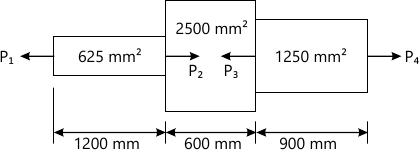10 M

2 (a) Derive an expression for volumetric strain of a rectangular bar, subjected to normal stress σ along its axis.
6 M
2 (b) Define 3 modulii of elasticity and write the relationship between them.
4 M
2 (c) A composite bar consisting of steel and aluminium components shown in fig Q2(c) is held firmly between two grips at the ends at a temperature of 60°C. Find the stresses in the two rods, when temperature falls to 20°C. If i) The ends do not yield ii) The ends yield by 0.25mm. Take Es=2×105 N/mm2
αs=1.17×10-5 /°C.
EA=0.7×105 N/mm2
αA=2.34×10-5 /°C.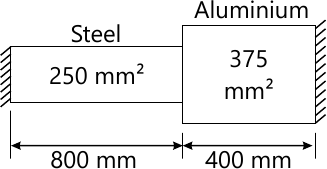10 M

3 (a) Define the principle stresses and principle planes.
3 M
3 (b) Explain the construction of Mohr's circle and represent principle stress.
7 M
3 (c) At a certain point in a strained material the stress condition shown in fig Q3(c) exists. Find i) The normal and shear stress on the inclined plane AB ii) Principle stresses and principle planes
iii) Maximum shear stress.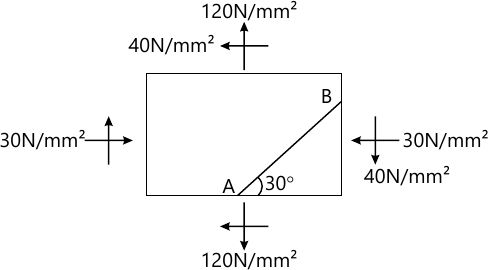10 M

4 (a) Derive an expression for strain energy, when a member subjected to impact loads.
6 M
4 (b) Derive an expression for circumferential stress of a thin cylinder.
4 M
4 (c) A C.I. pipe has 200mm internal diameter and 50mm metal thickness and carries water under a pressure of 5N/mm2. Calculate the maximum intensities of circumferential stress and sketch the distribution of circumferential stress intensity and intensity of radial pressure across the section.
10 M

5 (a) Establish relationship between distributed load, shear force and bending moment at a cross- section of a beam.
6 M
5 (b) For the beam shown in fig,Q5(b), draw SFD and BMD and mark the value at the salient points.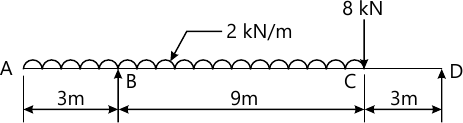14 M

6 (a) Prove that $$\dfrac {M}{I} = \dfrac {\sigma}{Y} = \dfrac {E} {R}$$ <\span> with usual notations.
10 M
6 (b) A beam of an I-section consist of 180mm×15mm flanges and a web of 280mm × 15mm thickness. It is subjected to a shear force of 60kN. Sketch the shear stress distribution along the depth of the section.
10 M

7 (a) Derive an expression $$EI\dfrac {d^2y} {dx^2}=M$$ <\span>, with usual notations.
10 M
7 (b) Determine the deflection at points C, D and E in the beam shown in fig Q7(b). Takes E=200 kN/mm2 and I=60×106mm4.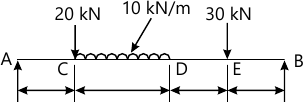10 M

8 (a) A hollow shaft of diameter ratio 3/8 is required to transmit 588KW at 110 rpm, the maximum torque being 120% of the mean. Shear stress is not to exceed 63N/mm2 and twist in a length of 3m not to exceed 1.4 degrees. Calculate external diameter of shaft which would satisfy these conditions. Take modulus of rigidity=84 Gpa.
12 M
8 (b) Define slenderness ratio and derive Euler's expression for buckling load for column with both ends hinged.
8 M

More question papers from Mechanics of Materials